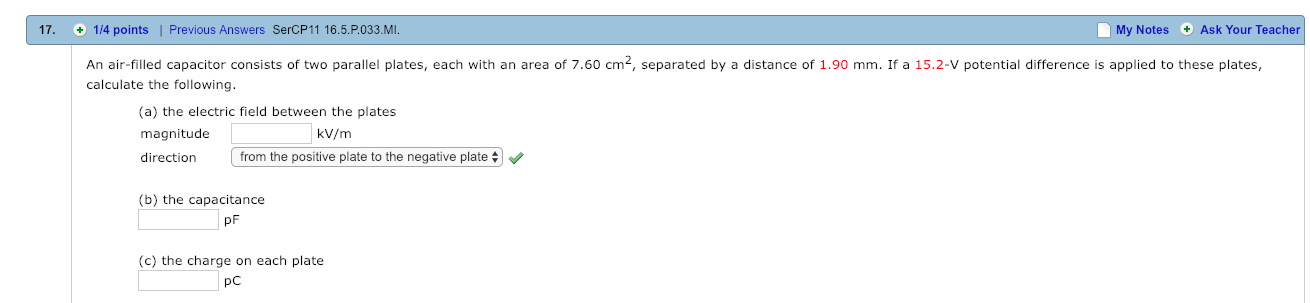# 17. 1/4 points| Previous Answers SerCP11 16.5.P.033.MI.points 1Previous Answers SercP11 16.5 P.033 MI..My Notes Ask Your TeacherAn air-filled capacitor consists of two parallel plates, each with an area of 7.60 cm, separated by a distance of 1.90 mm. If a 15.2-V potential difference is applied to these platescalculate the following.(a) the electric field between the platesmagnitudedirection from the positive plate to the negative platekV/m(b) the capacitancePF(c) the charge on each platepC

Question
3 viewshelp_outlineImage Transcriptionclose17. 1/4 points| Previous Answers SerCP11 16.5.P.033.MI. points 1Previous Answers SercP11 16.5 P.033 MI.. My Notes Ask Your Teacher An air-filled capacitor consists of two parallel plates, each with an area of 7.60 cm, separated by a distance of 1.90 mm. If a 15.2-V potential difference is applied to these plates calculate the following. (a) the electric field between the plates magnitude direction from the positive plate to the negative plate kV/m (b) the capacitance PF (c) the charge on each plate pC fullscreen
check_circle

star
star
star
star
star
1 Rating
Step 1

Given:      Area(A) = 7.60 cm2 = 0.00076m2

distance(d) = 1.90mm = 0.0019m

Potential difference = 15.2V

Find:           (a) Electric field(E) = ?

(b) the capacitance(C) = ?

(c) the charge on each plate(Q) = ?

Step 2

(a)   to calculate electric field, use the formula as follows:

Step 3

(b) to calculate capacitance, us...

### Want to see the full answer?

See Solution

#### Want to see this answer and more?

Solutions are written by subject experts who are available 24/7. Questions are typically answered within 1 hour.*

See Solution
*Response times may vary by subject and question.
Tagged in

### Electric Charges and Fields